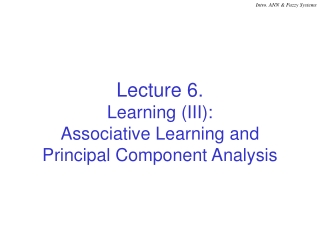DownloadDownload PresentationLecture 6. Learning (III): Associative Learning and Principal Component Analysis

# Lecture 6. Learning (III): Associative Learning and Principal Component Analysis

Télécharger la présentation## Lecture 6. Learning (III): Associative Learning and Principal Component Analysis

- - - - - - - - - - - - - - - - - - - - - - - - - - - E N D - - - - - - - - - - - - - - - - - - - - - - - - - - -
##### Presentation Transcript

1. Lecture 6.Learning (III):Associative Learning and Principal Component Analysis

2. Outline • Associative Learning: Hebbian Learning • Use Associative Learning to compute Principal Component (C) 2001 by Yu Hen Hu

3. Associative (Hebbian) Learning • A Learning rule postulated by Hebb (1949) on the associative learning at the cellular level. D wkj(n) = wkj(n+1)  wkj(n) =  dk(n)xi(n) or D w(n) =  dk(n)x(n) The change of synaptic weights is proportional to the correlation of the (desired) activation dk(n) and the input x(n). • The weight vector w(n) converges to the cross-correlation of dk(n) and x(n): (C) 2001 by Yu Hen Hu

4. Potential Applications of Associative Learning • Pattern Retrieval • Each pattern (input: x) is associated with a label (output: d). • The association information is stored in weights of a single layer network W. • When a test pattern is presented to the input, the label of a stored pattern that is closest to the test pattern should appear as the output. • This is the mechanism of an “associative memory” (C) 2001 by Yu Hen Hu

5. M pairs of training vectors {(bm, am); m = 1, 2, ..., M}. Weight matrix: Retrieval: y=Wx. Let x = bk, Linear Associative Memory (C) 2001 by Yu Hen Hu

6. Example • Consider 3 input patterns: x(1) = [1 2 –1]T, x(2) = [–1 1 1]T, x(3) = [1 0 1]T, and their corresponding 2D labels: y(1) = [–1 2]T, y(2) = [2 4]T, y(3) = [1 –3]T. • Compute the cross correlation matrix using the Hebbian learning,with learning rate  = 1: • Recall: Present x(2). Then y = W x(2) = [6 12]T = 3[2 4]T which is proportional to y(2)! (C) 2001 by Yu Hen Hu

7. Hebbian Learning Modifications • Problem with Hebbian learning: when both dk(n) and xj(n) are positive, the weight wkj(n) will grow unbound. • Kohonen (1988) proposed to add a forgetting factor: Dwkj(n) = dk(n) xj(n) – a dk(n) wkj(n) or Dwkj(n) = adk(n)[c xj(n) – wkj(n)]; where c = h/a • Analysis: Suppose that xj(n) > 0 and dk(n) > 0. When wkj(n) increases until c xj(n) < wkj(n), Dwkj(n) < 0. This, in turn, will reduces wkj(n) for future n. • It can be shown that when |adk(n)| < 1, |wkj(n)| will be bounded if dk(n) and xj(n) are bounded. (C) 2001 by Yu Hen Hu

8. Principal Component Analysis (PCA) • Pricipal component: part of signal (feature vectors) which consists of most energy of the signal! • Also known as Karhunen-Loeve Transformation • A linear transform of n-dim vector x, Tx such that ||x–Tx||2 is minimum among all n by n matrices T whose rank = p < n. • Applications: Feature dimension reduction Data Compression (C) 2001 by Yu Hen Hu

9. PCA Method Given {x(k); 1  k  K}. Let dimension of x(k) = n. 1. Form a n by n Covariance matrix estimate 2. Eigenvalue decomposition 3. Principal components y(k) = VTpx(k) = [v1v2 … vp]Tx(k), p  n. Tx(k) = Vpy(k) = VpVTpx(k) (C) 2001 by Yu Hen Hu

10. Optimality of Principal Components • Among all n by n matrices T' whose rank = p  n, || x – Tx ||2= li || x – T'x ||2 This is the orthogonality principle: • y(k) is the p-dimensional representation of x in the subspace spanned by columns of Vp. || y(k)|| = || Tx(k)|| is the length of the shadow of x(k). (C) 2001 by Yu Hen Hu

11. y1 x1  y2 x2        yN xM Principal Component Network • Linear neuron model: Choose w to maximize E{||y||2} = wTE{xxT}w = wTCw subject to ||w||2 = 1. • Generalized Hebbian learning rule: ghademo.m, ghafun.m (C) 2001 by Yu Hen Hu